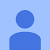# Simple Matrix Factorization example on the Movielens dataset using Pyspark

Matrix factorization works great for building recommender systems. I think it got pretty popular after the Netflix prize competition. All you need to build one is information about which user bought or rated which items and you’re good to go. And I was surprised how amazingly simple to build one with Pyspark ML libraries. So I’ll demonstrate how to code one up quickly using RDDs and DataFrames separately.

I’ll mostly focus on building the model in this tutorial. If you’re interested in learning more about Matrix factorization or Singular Value Decomposition, there are some amazing resources out there. …

# Basic data preparation in Pyspark — Capping, Normalizing and Scaling

In this blog, I’ll share some basic data preparation stuff I find myself doing quite often and I’m sure you do too. I’ll use Pyspark and I’ll cover stuff like removing outliers and making your distributions normal before you feed your data into any model, be it linear regression or nearest neighbour searches.

You don’t always need to remove outliers and skewness from your data. It highly depends on how you’re going to use it. For example, algorithms like decision trees arnt affected by outliers, but algorithms like linear regression or even neural nets expect your data to have somewhat normal distributions. Scaling also has a big effect on any mode which calculates distances between observations. I have noticed pretty distinct jumps in model performance before and after removing skewness from my data in some projects. …

# Visualising Indian startup investments using Python — violin plots, heatmaps and sankey diagrams

Sometimes histograms and scatterplots arnt enough. Here I’ll cover some of the more complicated plots that you might need to use — violin plots, heatmaps and sankey diagrams. I’ll mostly use python and I’ve picked up this data from here, its data on the startup investment scene in India. The dataset has Indian startup funding information between January 2015 and August 2017.

First I’ll read the data and do some cleaning.

`# importing some stuffimport pandas as pdimport numpy as npimport mathfrom datetime import datetime# importing tsuff for plottingimport seaborn as snsfrom matplotlib import pyplot as pltfrom pylab import…`

# Topic modelling with Latent Dirichlet Allocation (LDA) in Pyspark

In one of the projects that I was a part of we had to find topics from millions of documents. You can try doing topic modelling using two methods. Do Non negative Matrix Factorization (NMF) or LDA. NMF is supposed to be a lot faster than LDA, but LDAs supposed to be more accurate. Problem is LDA takes a long time, unless you’re using distributed computing. Thats why I wanted to show you how you approach this problem using Spark in python.

I’m using a random table whose one columns has some sort of reviews for fashion items. …

# Recommender system on the Movielens dataset using an Autoencoder and Tensorflow in Python

I’m a huge fan of autoencoders. They have a ton of uses. They can be used for dimensionality reduction like I show here, they can be used for image denoising like I show in this tutorial and a lot of other stuff.

Today I’ll use it to build a recommender system using the movielens 1 million dataset. You can download it yourself from here. I was mostly inspired by this research paper to build this model. First let me show you what the neural net model will look like. I took this pic straight out of the research paper.

This is a shallow neural net with only one hidden layer. So I’ll just feed in all the movie ratings watched by a user and expect a more generalized rating distribution per user to come out. I can use that to get an idea of what their ratings would be for movies they havn’t watched. …

# Denoising MNIST images using an Autoencoder and Tensorflow in python

Previously I had written sort of a tutorial on building a simple autoencoder in tensorflow. In that tutorial I had used the autoencoder for dimensionality reduction. Check it out if you want to. It has a much more detailed explanation on how to build the autoencoder itself. Here, I’ll use the exact same model to show another use of autoencoders — denoising images.

So let’s get started. I’ll use the famous MNIST handwriting data here.

`# Importing tensorflowimport tensorflow as tf# importing the datafrom tensorflow.examples.tutorials.mnist import input_data# Importing some more librariesimport matplotlib.pyplot as pltfrom numpy import loadtxtimport numpy as npfrom pylab import…`

# Simple Autoencoder example using Tensorflow in Python on the Fashion MNIST dataset

Autoencoders can be used to solve a lot of problems. The one I’ll try to solve here is that of dimensionality reduction. This is a pretty common problem in data science. I’ve seen it pop up in a lot of projects that I’ve worked on. If you have structured data, usually how you’d deal with it is use PCA, SVD etc. But here I’ll use an autoencoder to get latent features for every image.

In this tutorial, I’ll focus more on building a simple tensorflow model. You can build it using keras too. Their website has some really helpful examples of doing just that. …